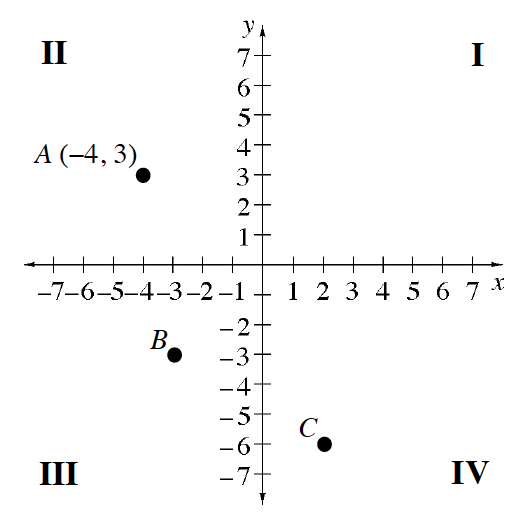### Home > CC1MN > Chapter 3 > Lesson 3.2.3 > Problem3-122

3-122.

One of the topics you will review in this course is reading graphs. Look at the graph at right. This graph shows positive and negative values on both axes. It divides the plane into four parts, or quadrants. It is called a four-quadrant graph. The quadrants are numbered I, II, III, and IV in a counter-clockwise manner as shown.

1. The coordinates (the $x$- and $y$- values) for point $A$ are $(-4,3)$. Explain how these numbers tell you the position of point $A$ using the graph.

Remember that the $x$-axis is the horizontal axis, and the $y$-axis is the vertical axis. The $x$- and $y$-coordinates correspond to locations on these axes.

2. Name the coordinates $(x,y)$ for points $B$ and $C$.

Point B is $(−3,−3)$since it is $3$ units left of the origin and $3$ units below it. Use this to help name point $C$.

1. If Deepak moved from point $A$ $8$ units to the right and $10$ units down, at what point on the graph would he end up? Which quadrant is the new point in?

• Label the new point $A′$.

This new point is in the fourth quadrant (labelled IV), since the $x$-coordinate is positive and the $y$-coordinate is negative.